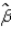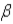Question 3
Multiple Choice

# Ifj, an unbiased estimator ofj, is also a consistent estimator ofj, then when the sample size tends to infinity: A)the distribution ofj collapses to a single value of zero. B)the distribution ofj diverges away from a single value of zero. C) the distribution ofj collapses to the single pointj. D) the distribution ofj diverges away fromj.

C Explanation: Ifj, an unbiased estimator ofj, is also a consistent estimator ofj, then when the sample size tends to infinity the distribution ofj collapses to the single pointj.